# C++ Program For Deleting A Node In A Doubly Linked List

• Last Updated : 15 Dec, 2021

Pre-requisite: Doubly Link List Set 1| Introduction and Insertion

Write a function to delete a given node in a doubly-linked list.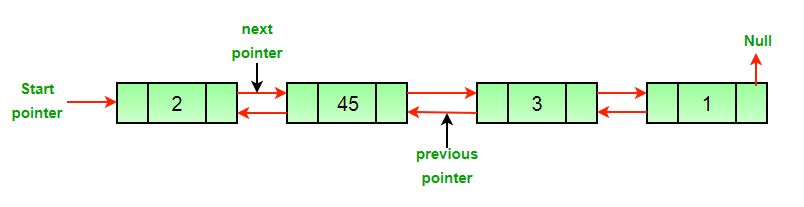Approach: The deletion of a node in a doubly-linked list can be divided into three main categories:

• After the deletion of the head node.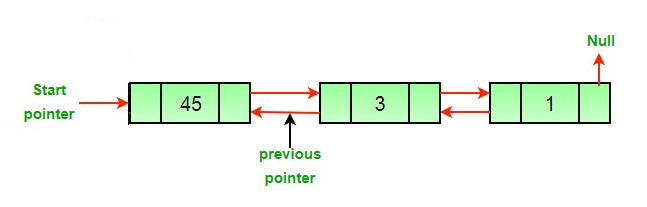• After the deletion of the middle node.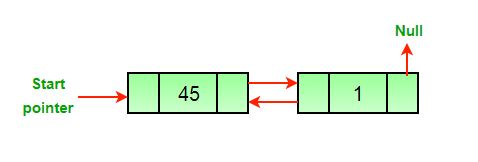• After the deletion of the last node.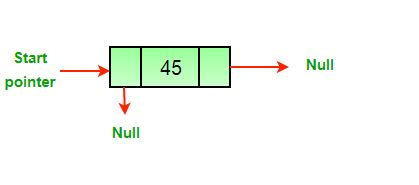All three mentioned cases can be handled in two steps if the pointer of the node to be deleted and the head pointer is known.

1. If the node to be deleted is the head node then make the next node as head.
2. If a node is deleted, connect the next and previous node of the deleted node.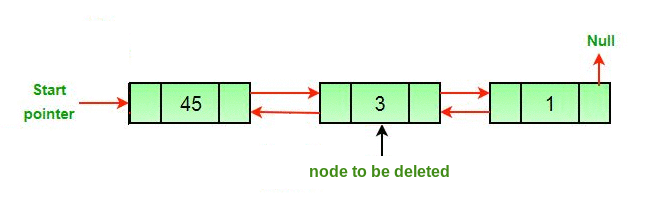Algorithm

• Let the node to be deleted be del.
• If node to be deleted is head node, then change the head pointer to next current head.
```if headnode == del then
• Set next of previous to del, if previous to del exists.
```if del.nextNode != none
del.nextNode.previousNode = del.previousNode ```
• Set prev of next to del, if next to del exists.
```if del.previousNode != none
del.previousNode.nextNode = del.next```

## C++

 `// C++ program to delete a node from``// Doubly Linked List``#include ``using` `namespace` `std;`` ` `// Anode of the doubly linked list``class` `Node ``{ ``    ``public``:``    ``int` `data; ``    ``Node* next; ``    ``Node* prev; ``}; `` ` `/* Function to delete a node in a Doubly ``   ``Linked List. head_ref --> pointer to``   ``head node pointer. del --> pointer to ``   ``node to be deleted. */``void` `deleteNode(Node** head_ref, Node* del) ``{ ``    ``// Base case ``    ``if` `(*head_ref == NULL || del == NULL) ``        ``return``; `` ` `    ``// If node to be deleted is head node ``    ``if` `(*head_ref == del) ``        ``*head_ref = del->next; `` ` `    ``/* Change next only if node to be ``       ``deleted is NOT the last node */``    ``if` `(del->next != NULL) ``        ``del->next->prev = del->prev; `` ` `    ``/* Change prev only if node to be ``       ``deleted is NOT the first node */``    ``if` `(del->prev != NULL) ``        ``del->prev->next = del->next; `` ` `    ``/* Finally, free the memory occupied ``       ``by del*/``    ``free``(del); ``    ``return``; ``} `` ` `// UTILITY FUNCTIONS ``/* Function to insert a node at the``   ``beginning of the Doubly Linked List */``void` `push(Node** head_ref, ``int` `new_data) ``{ ``    ``// Allocate node ``    ``Node* new_node = ``new` `Node();`` ` `    ``// Put in the data ``    ``new_node->data = new_data; `` ` `    ``/* Since we are adding at the ``       ``beginning, prev is always NULL */``    ``new_node->prev = NULL; `` ` `    ``/* Link the old list off the ``       ``new node */``    ``new_node->next = (*head_ref); `` ` `    ``/* Change prev of head node to ``       ``new node */``    ``if` `((*head_ref) != NULL) ``        ``(*head_ref)->prev = new_node; `` ` `    ``/* Move the head to point to the ``       ``new node */``    ``(*head_ref) = new_node; ``} `` ` `/* Function to print nodes in a given ``   ``doubly linked list. This function is ``   ``same as printList() of singly linked list */``void` `printList(Node* node) ``{ ``    ``while` `(node != NULL) ``    ``{ ``        ``cout << node->data << ``" "``; ``        ``node = node->next; ``    ``} ``} `` ` `// Driver code``int` `main() ``{ ``    ``// Start with the empty list ``    ``Node* head = NULL; `` ` `    ``/* Let us create the doubly linked list ``       ``10<->8<->4<->2 */``    ``push(&head, 2); ``    ``push(&head, 4); ``    ``push(&head, 8); ``    ``push(&head, 10); `` ` `    ``cout << ``"Original Linked list "``; ``    ``printList(head); `` ` `    ``/* Delete nodes from the doubly ``       ``linked list */``    ``// Delete first node``    ``deleteNode(&head, head); `` ` `    ``// Delete middle node``    ``deleteNode(&head, head->next); `` ` `    ``// Delete last node``    ``deleteNode(&head, head->next); `` ` `    ``/* Modified linked list will be ``       ``NULL<-8->NULL */``    ``cout << ``"Modified Linked list "``; ``    ``printList(head); `` ` `    ``return` `0;``} ``// This code is contributed by rathbhupendra`

Output:

```Original Linked list 10 8 4 2

Complexity Analysis:

• Time Complexity: O(1).
Since traversal of the linked list is not required so the time complexity is constant.
• Space Complexity: O(1).
As no extra space is required, so the space complexity is constant.

Please refer complete article on Delete a node in a Doubly Linked List for more details!

My Personal Notes arrow_drop_up